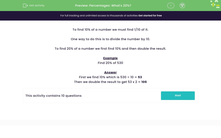# Percentages: What's 20%?

In this worksheet, students understand how to calculate 20% of a given number.Key stage:  KS 2

Curriculum topic:   Number: Fractions, Decimals and Percentages

Curriculum subtopic:   Recognise Percentages

Popular topics:   Percentages worksheets

Difficulty level:#### Worksheet Overview

To find 10% of a number we must find 1/10 of it.

One way to do this is to divide the number by 10.

To find 20% of a number we first find 10% and then double the result.

Example

Find 20% of 530

First we find 10% which is 530 ÷ 10 = 53

Then we double the result to get 53 x 2 = 106

### What is EdPlace?

We're your National Curriculum aligned online education content provider helping each child succeed in English, maths and science from year 1 to GCSE. With an EdPlace account you’ll be able to track and measure progress, helping each child achieve their best. We build confidence and attainment by personalising each child’s learning at a level that suits them.

Get started